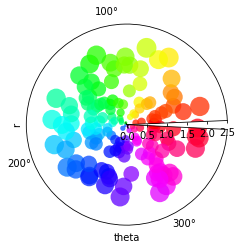Polar scatter demo¶

In :
import numpy as np
import holoviews as hv
from holoviews import dim

hv.extension('matplotlib')Define data¶

In :
# Compute areas and colors
N = 150
r = 2 * np.random.rand(N)
theta = 2 * np.pi * np.random.rand(N)
colors = theta

scatter = hv.Scatter((theta, r), 'theta', 'r').redim(r=dict(range=(0,2.5)))

Plot¶

In :
scatter.opts(
alpha=0.75, color='theta', cmap='hsv', projection='polar', s=dim('r')*200)
Out: